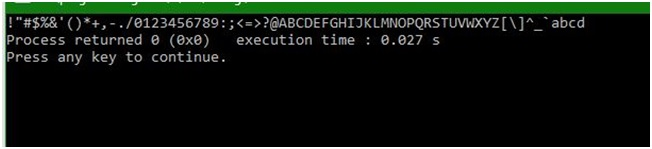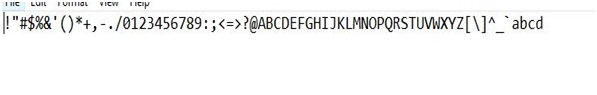# putc() function of stdio.h in C

In this article, we are going to learn about the putc() function of stdio.h header file in C programming language, and use it to put characters inside a file with the help of file pointer.
Submitted by Abhishek Sharma, on April 12, 2018

If you are handling files in your application then coping one file into other or from the same file will something you will encounter. Therefore, using the function putc() will help you doing this.

The Function is use to write a single character in file. The Function needs only two parameters first a character and second is the file itself.

Example:

```    putc('a', FILE);
//Here, FILE is the file variable.

Output:
It will write 'a' on the file at the current
position of the cursor.
```

## stdio.h - putc() function Example in C

```#include <stdio.h>

int main ()
{
// initializing the type of variables
FILE *F;
int c;

// opening the file in write mode
F = fopen("abc.txt", "w");
for( c = 33 ; c <= 100; c++ ) {
putc(c, F);
}
fclose(F);

// opening the file in read mode
F = fopen("abc.txt","r");
while(1) {
c = fgetc(F);
if( feof(F) ) {
break ;
}
// printing on console
printf("%c", c);
}

fclose(F);

return(0);
}
```

OutputFile's content